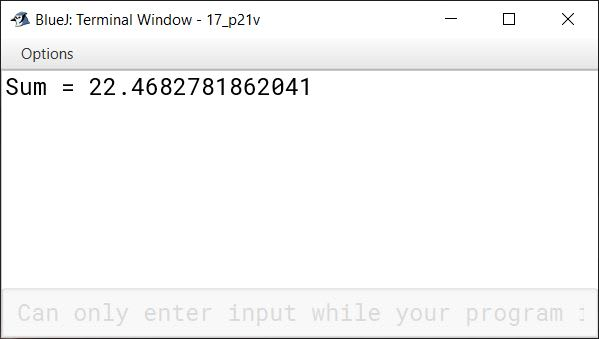# Iterative Constructs in Java

## Multiple Choice Questions

#### Question 1

Which of the following loop executes at least once?

1. for
2. while
3. do-while
4. None of these

do-while

Reason — do-while loop is an exit controlled loop, i.e., it checks the condition at the exit point. Therefore, it always executes at least once.

#### Question 2

Which of the following is an exit-controlled loop?

1. for
2. do-while
3. while
4. None of these

do-while

Reason — do-while is an exit-controlled loop.

#### Question 3

Which of the following is an invalid loop?

1. repeat
2. while
3. do-while
4. for

repeat

Reason — repeat is not a Java keyword. To repeat a certain action in Java, we use for, while and do-while loops.

#### Question 4

Which of the following is not a jump statement in Java?

1. return
2. jump
3. break
4. continue

jump

Reason — return, continue and break are jump statements in Java.

#### Question 5

How many times will the following code print "Hello"?

```for (int i = 1; i <= 5; i++);
{
System.out.println("Hello");
}```
1. 0
2. 1
3. 5
4. 4

1

Reason — The for loop has a semicolon `;` at the end. Thus, it becomes an empty loop and the program just waits till the loop finishes. Then the program executes the next statement `System.out.println("Hello");` and prints `Hello` on the output screen.

#### Question 6

What will be the output of the following code?

```public static void main(String args[])
{
int sum = 0;
for (int i= 1; i <= 5; i++)
{
sum = i;
}
System.out.println(sum);
}```
1. 15
2. 21
3. 5
4. 0

5

Reason — The values of i and sum as per the execution of the for loop are as follows:

No. of IterationsValue of iValue of sumTest Condition
1st11True
2nd22True
3rd33True
4th44True
5th55False

Thus, for loop terminates when the value of `sum` is 5. Hence the output is 5.

#### Question 7

How many times will the following loop execute?

```public static void main(String args[])
{
int sum = 0;
for (int i = 10; i > 5; i++)
{
sum += i;
}
System.out.println(sum);
}```
1. 5
2. 0
3. 15
4. Infinite loop

Infinite loop

Reason — The loop will run for infinite times as `i` is initialised as `10` and the update expression `i++` increments i after each iteration. Hence, the test expression `i > 5` will always remain true.

#### Question 8

How many times will the following loop execute?

```public static void main(String args[])
{
int i = 1;
while (i < 10)
if (i++ % 2 == 0)
System.out.println(i);
}```
1. 4
2. 5
3. 0
4. 10

Loop executes 9 times

Reason — There is a misprint in the book. The loop executes 9 times. Value of `i` is printed 4 times inside the loop. The condition `if (i++ % 2 == 0)` will be `false` when `i` is odd and `true` when `i` is even. Value of `i` will be incremented after if check is performed due to post increment opertor `i++`. Execution of the loop is summarized in the table below:

Iterationsi`i++ % 2 == 0`OutputRemarks
1st1False Value of `i` becomes 2 after if check as `i++` increments it after the evaluation of `i++ % 2 == 0`
2nd2True3`i` becomes 3 after if check, hence 3 is printed in this iteration.
3rd3False `i` becomes 4.
4th4True5`i` becomes 5.
5th5False `i` becomes 6.
6th6True7`i` becomes 7.
7th7False `i` becomes 8.
8th8True9`i` becomes 9.
9th9False `i` becomes 10.

As value of `i` is now 10, the test condition of while loop becomes `false` hence, 10th iteration of the loop is not executed.

## State whether the given statements are True or False

#### Question 1

To execute a do-while loop, the condition must be true in the beginning.
False

#### Question 2

The while loop is an exit-controlled loop.
False

#### Question 3

The while part of a do-while statement must be terminated by a semicolon.
True

#### Question 4

The continue statement terminates the current loop and then continues from the statement immediately following the current loop.
False

#### Question 5

The return statement is a jump statement.
True

#### Question 6

The for loop may contain multiple initialisations and updates.
True

#### Question 7

A loop that never terminates is called an empty loop.
False

#### Question 8

The do-while loop executes at least once even if the condition is false.
True

## Assignment Questions

#### Question 1

What are looping control structures?

A loop is a set of instructions that is continually repeated until a certain condition is met. Looping control structures refer to certain looping constructs which execute a block of code repeatedly until a certain condition remains true. For example, for loop, while loop, do - while loop etc.

#### Question 2

What are the essential parts of a looping control structure?

The essential parts of a looping control structure are as follows:

1. Initialisation — This segment initialises the loop control variable before starting the loop. It is executed only once at the beginning of the loop.
For example, `int counter = 1;`
2. Test-condition — The test-condition is the expression that is evaluated at the beginning of each iteration. Its value determines whether the body of the loop is to be executed (test condition is true) or the loop is to be terminated (test condition is false).
For example, `counter <= 10`
3. Update — This is the increment or decrement operation of the control variable. This operation is performed at the end of each iteration.
For example, `counter++ ;`

#### Question 3

How are these statements different from each other:

(i) break

(ii) continue

(iii) System.exit(0)

(i) The break statement terminates the current loop or switch statement. The execution then continues from the statement immediately following the current loop or switch statement.

(ii) The continue statement tells the computer to skip the rest of the current iteration of the loop. However, instead of jumping out of the loop completely like break statement, it jumps back to the beginning of the loop and continues with the next iteration. This includes the evaluation of the loop controlling condition to check whether any further iterations are required.

(iii) Unlike break and continue statements which are used to control the flow of execution, System.exit(0) command terminates the execution of the program by stopping the Java Virtual Machine which is executing the program. It is generally used when due to some reason it is not possible to continue with the execution of the program.

#### Question 4(i)

Identify all the errors in the following repetitive statements.

```for	(int i = 5; i > 0; i++)
{
System.out.println("Java");
}```

The test expression `i > 0` will always remain `true` and hence, the for loop will become an infinite loop and keep on iterating.

#### Question 4(ii)

Identify all the errors in the following repetitive statements.

```while (z < 1 && z > 100)
{
a = b;
}```

The test expression `z < 1 && z > 100` will never be `true` as the conditions `z < 1` and `z > 100` cannot be `true` at the same time. Thus, the `&&` operator will always result in `false`.

#### Question 4(iii)

Identify all the errors in the following repetitive statements.

```while (x == y)
{
xx = yy;
x = y;
}```

The test expression `x == y` will always remain `true` as in each iteration `x = y` is executed, which will store the value of `y` in `x`. Thus, an infinite loop will be generated.

#### Question 5

What is an empty statement? Explain its usefulness.

Empty statement consists only of a semicolon `;`. It is useful when we want to make a loop an empty loop.

To make a for loop an empty loop, we write the following code:

`for (int i = 1 ; i <=10 ; i ++);`

#### Question 6

Convert the following for loop statement into the corresponding while loop and do-while loop:

```int	sum = 0;
for	(int i= 0; i <= 100; i++)
sum = sum + i;```

while loop

```int sum = 0, i = 0;
while (i <= 100)    {
sum = sum + i;
i++;
}```

do-while loop

```int sum = 0, i = 0;
do {
sum = sum + i;
i++;
} while(i <= 100);```

#### Question 7

What are the differences between while loop and do-while loop?

do-while loopwhile loop
do-while is an exit-controlled loop.while is an entry-controlled loop.
do-while loop checks the test condition at the end of the loop.while loop checks the test condition at the beginning of the loop.
do-while loop executes at least once, even if the test condition is false.while loop executes only if the test condition is true.
do-while loop is suitable when we need to display a menu to the user.while loop is helpful in situations where number of iterations is not known.

#### Question 8(i)

How many times are the following loop bodies repeated? What is the final output in each case?

```int x = 1;
while (x < 10)
if(x % 2 == 0)
System.out.println(x);```

The loop repeats for infinite times.

##### Output

The given code gives no output.

##### Explanation

The value of `x` is `1`. The test condition of while loop — `x < 10` is `true` but the test condition of if — `x % 2 == 0` is `false`. In the absence of update expression, the while loop continues infinitely.

#### Question 8(ii)

How many times are the following loop bodies repeated? What is the final output in each case?

```int y = 1;
while (y < 10)
if (y % 2 == 0)
System.out.println(y++);```

The loop repeats for infinite times.

##### Output

The given code gives no output.

##### Explanation

The value of `y` is `1`. The test condition of while loop — `y < 10` is `true` but the test condition of if — `y % 2 == 0` is `false`. Thus, the control does not enter the if statement and the value of `y` remains `1` infinitely. Thus, the while loop continues infinitely.

#### Question 8(iii)

How many times are the following loop bodies repeated? What is the final output in each case?

```int	z = 1;
while (z < 10)
if((z++) % 2 == 0)
System.out.println(z);```

The loop executes 9 times.

##### Output
``````3
5
7
9
``````
##### Explanation

The loop executes 9 times. Value of `z` is printed 4 times inside the loop. The condition `if((z++) % 2 == 0)` will be false when `z` is odd and true when `z` is even. Value of `z` will be incremented after if check is performed due to post increment operator `z++`. Execution of the loop is summarized in the table below:

Iterationsz`(z++) % 2 == 0`OutputRemarks
1st1False Value of `z` becomes 2 after if check as `z++` increments it after the evaluation of `(z++) % 2 == 0`
2nd2True3`z` becomes 3 after if check, hence 3 is printed in this iteration.
3rd3False `z` becomes 4.
4th4True5`z` becomes 5.
5th5False `z` becomes 6.
6th6True7`z` becomes 7.
7th7False `z` becomes 8.
8th8True9`z` becomes 9.
9th9False `z` becomes 10.

As value of `z` is now 10, the test condition of while loop becomes `false` hence, 10th iteration of the loop is not executed.

#### Question 9

Write a program to accept n number of input integers and find out:

i. Number of positive numbers

ii. Number of negative numbers

iii. Sum of positive numbers

```import java.util.Scanner;

public class KboatIntegers
{
public static void main(String args[]) {
Scanner in = new Scanner(System.in);
int pSum = 0, pCount = 0, nCount = 0;
System.out.print("Enter the number of integers: ");
int num = in.nextInt();

System.out.println("Enter " + num + " integers: ");
for (int i = 1; i <= num; i++) {
int n = in.nextInt();
if (n >= 0) {
pSum += n;
pCount++;
}
else
nCount++;
}

System.out.println("Positive numbers = " + pCount);
System.out.println("Negative numbers = " + nCount);
System.out.println("Sum of positive numbers  = " + pSum);
}
}```
##### Output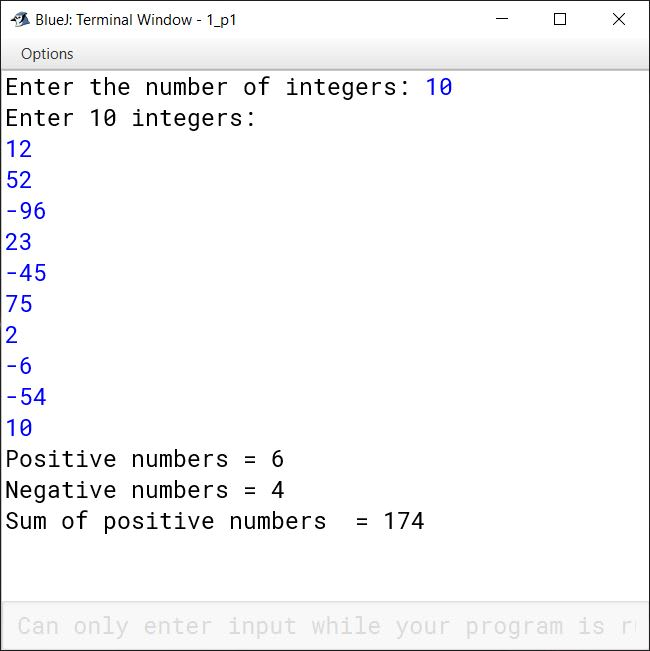#### Question 10

Write a program using do-while loop to compute the sum of the first 50 positive odd integers.

```public class KboatSumOdd
{
public static void main(String args[]) {
long sumOdd = 0;
int i = 1;
do {
sumOdd += i;
i += 2;
} while(i <= 50);

System.out.println("Sum of 50 odd positive numbers = " + sumOdd);
}
}```
##### Output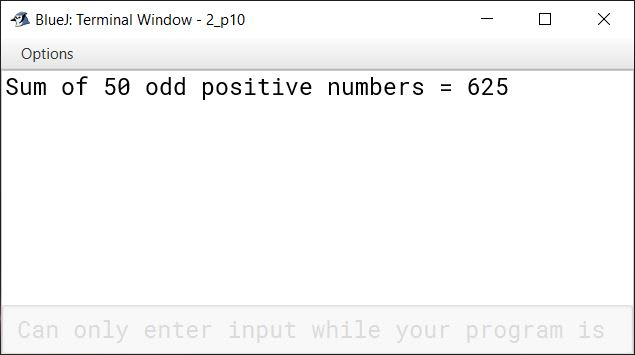#### Question 11

Write a program to read the number n via the Scanner class and print the Tribonacci series:

0, 0, 1, 1, 2, 4, 7, 13, 24, 44, 81 ...and so on.

Hint: The Tribonacci series is a generalisation of the Fibonacci sequence where each term is the sum of the three preceding terms.

```import java.util.Scanner;

public class KboatTribonacci
{
public static void main(String args[]) {
Scanner in = new Scanner(System.in);
System.out.print("Enter no. of terms : ");
int n = in.nextInt();

if(n < 3)
System.out.print("Enter a number greater than 2");
else    {
int a = 0, b = 0, c = 1;
System.out.print(a + " " + b + " " + c);

for (int i = 4; i <= n; i++) {
int term = a + b + c;
System.out.print(" " + term);
a = b;
b = c;
c = term;
}
}
}
}```
##### Output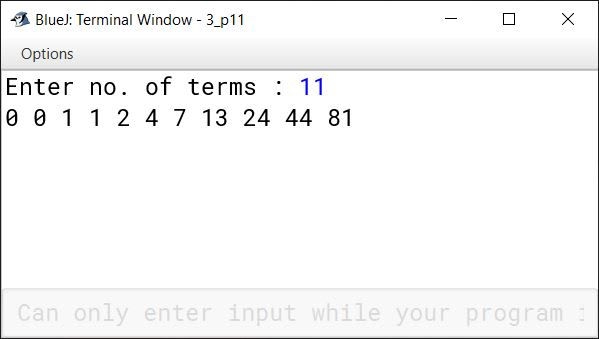#### Question 12

Write a program to calculate the value of Pi with the help of the following series:

Pi = (4/1) - (4/3) + (4/5) - (4/7) + (4/9) - (4/11) + (4/13) - (4/15) ...

Hint: Use while loop with 100000 iterations.

```public class KboatValOfPi
{
public static void main(String args[]) {
double pi = 0.0d, j = 1.0d;
int i = 1;
while(i <= 100000)  {
if(i % 2 == 0)
pi -= 4/j;
else
pi += 4/j;
j += 2;
i++;
}
System.out.println("Pi = " + pi);

}
}```
##### Output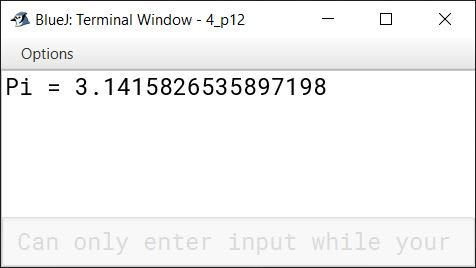#### Question 13

Write a menu-driven program to display the pattern as per user's choice:

Pattern 1       Pattern 2
ABCDE          B
ABCD            LL
ABC              UUU
AB                EEEE
A

For an incorrect option, an appropriate error message should be displayed.

```import java.util.Scanner;

{
public static void main(String args[]) {
Scanner in = new Scanner(System.in);
System.out.println("Enter 1 for pattern 1");
System.out.println("Enter 2 for Pattern 2");
int choice = in.nextInt();
switch (choice) {
case 1:
for (int i = 69; i >= 65; i--) {
for (int j = 65; j <= i; j++) {
System.out.print((char)j);
}
System.out.println();
}
break;

case 2:
String word = "BLUE";
int len = word.length();
for(int i = 0; i < len; i++) {
for(int j = 0; j <= i; j++) {
System.out.print(word.charAt(i));
}
System.out.println();
}
break;

default:
System.out.println("Incorrect choice");
break;

}
}
}```
##### Output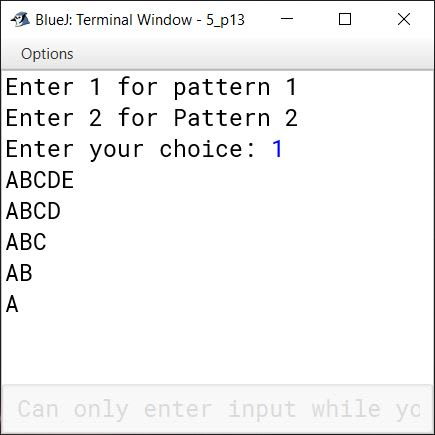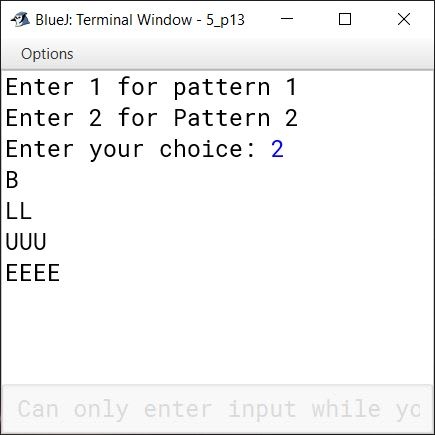#### Question 14

Write a program to input a number and check and print whether it is a Pronic number or not. (Pronic number is a number which is the product of two consecutive integers.)

Example: 12 = 3 x 4
20 = 4 x 5
42 = 6 x 7

```import java.util.Scanner;

public class KboatPronicNumber
{
public static void main(String args[]) {
Scanner in = new Scanner(System.in);
System.out.print("Enter the number to check: ");
int num = in.nextInt();

boolean isPronic = false;

for (int i = 1; i <= num - 1; i++) {
if (i * (i + 1) == num) {
isPronic = true;
break;
}
}

if (isPronic)
System.out.println(num + " is a pronic number");
else
System.out.println(num + " is not a pronic number");
}
}```
##### Output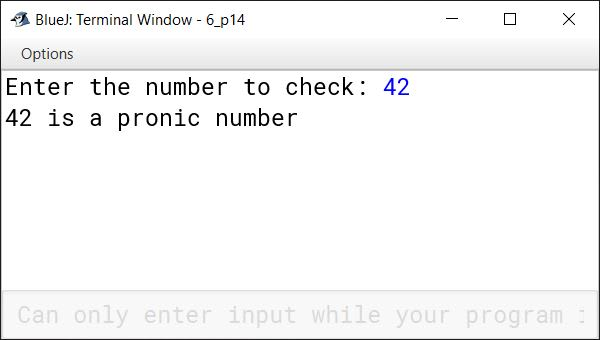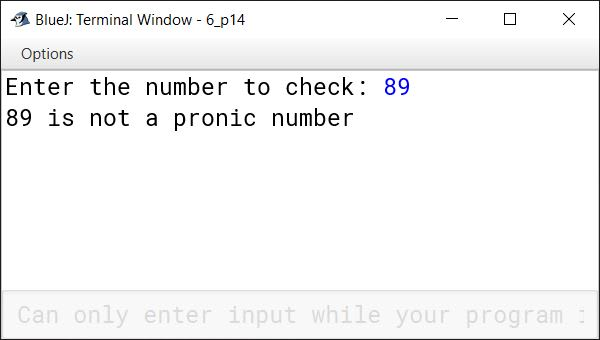#### Question 15

Write a program to accept a number and check and display whether it is a spy number or not. (A number is spy if the sum of its digits equals the product of the digits.)
Example: Consider the number, 1124. Sum of the digits = 1 + 1 + 2 + 4 = 8.
Product of the digits = 1 * 1 * 2 * 4 = 8.

```import java.util.Scanner;

public class KboatSpyNumber
{
public static void main(String args[]) {

Scanner in = new Scanner(System.in);

System.out.print("Enter Number: ");
int num = in.nextInt();

int digit, sum = 0;
int orgNum = num;
int prod = 1;

while (num > 0) {
digit = num % 10;

sum += digit;
prod *= digit;
num /= 10;
}

if (sum == prod)
System.out.println(orgNum + " is Spy Number");
else
System.out.println(orgNum + " is not Spy Number");

}
}```
##### Output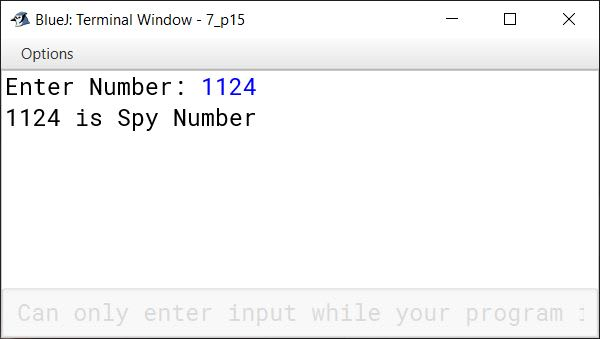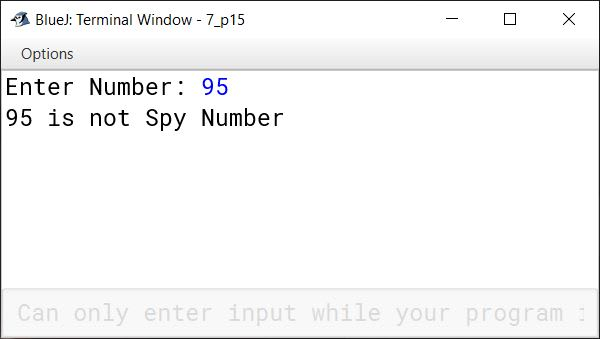#### Question 16

Write a program to accept a number and check and display whether it is a Niven number or not. (Niven number is a number which is divisible by the sum of its digits).
Example:
Consider the number 126.
Sum of its digits is 1 + 2 + 6 = 9 and 126 is divisible by 9.

```import java.util.Scanner;

public class KboatNivenNumber
{
public static void main(String args[]) {
Scanner in = new Scanner(System.in);
System.out.print("Enter number: ");
int num = in.nextInt();
int orgNum = num;

int digitSum = 0;

while (num != 0) {
int digit = num % 10;
num /= 10;
digitSum += digit;
}

/*
* digitSum != 0 check prevents
* division by zero error for the
* case when users gives the number
* 0 as input
*/
if (digitSum != 0 && orgNum % digitSum == 0)
System.out.println(orgNum + " is a Niven number");
else
System.out.println(orgNum + " is not a Niven number");
}
}```
##### Output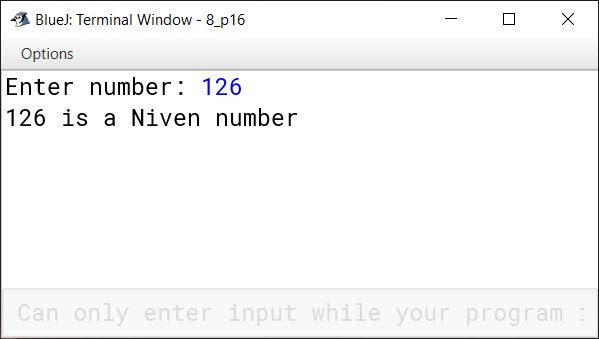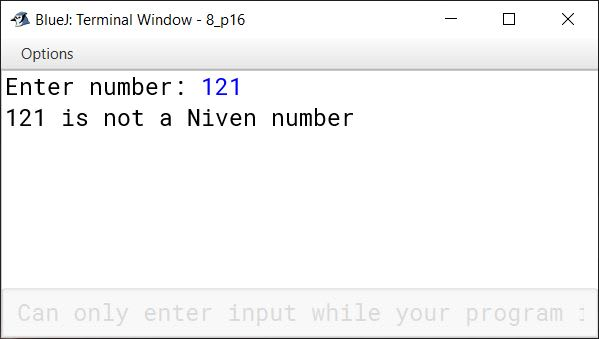#### Question 17

Using the switch statement, write a menu driven program to:

i. Generate and display the first 10 terms of the Fibonacci series 0, 1, 1, 2, 3, 5...
The first two Fibonacci numbers are 0 and 1, and each subsequent number is the sum of the previous two.

ii. Find the sum of the digits of an integer that is input.
Sample Input: 15390
Sample Output: Sum of the digits = 18
For an incorrect choice, an appropriate error message should be displayed.

```import java.util.Scanner;

public class KboatFibonacciNDigitSum
{
public static void main(String args[]) {
Scanner in = new Scanner(System.in);
System.out.println("1. Fibonacci Series");
System.out.println("2. Sum of digits");
int ch = in.nextInt();

switch (ch) {
case 1:
int a = 0, b = 1;
System.out.print(a + " " + b);
/*
* i is starting from 3 below
* instead of 1 because we have
* already printed 2 terms of
* the series. The for loop will
* print the series from third
* term onwards.
*/
for (int i = 3; i <= 10; i++) {
int term = a + b;
System.out.print(" " + term);
a = b;
b = term;
}
break;

case 2:
System.out.print("Enter number: ");
int num = in.nextInt();
int sum = 0;
while (num != 0) {
sum += num % 10;
num /= 10;
}
System.out.println("Sum of Digits " + " = " + sum);
break;

default:
System.out.println("Incorrect choice");
break;
}
}
}```
##### Output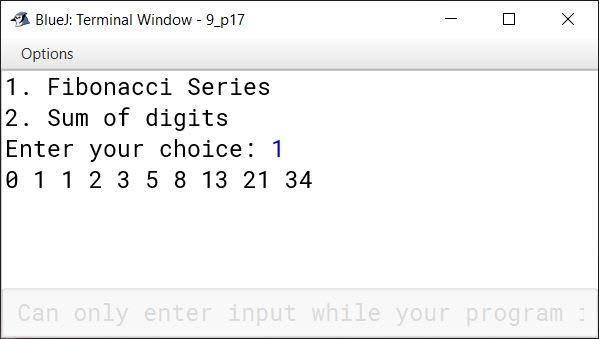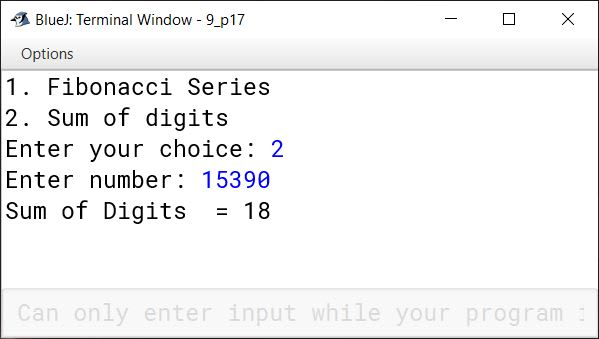#### Question 18

Write a program to input a number and print whether the number is a special number or not. (A number is said to be a special number, if the sum of the factorial of the digits of the number is same as the original number).

```import java.util.Scanner;

public class KboatSpecialNum
{
public static void main(String args[]) {
Scanner in = new Scanner(System.in);
System.out.print("Enter number: ");
int num = in.nextInt();

int t = num;
int sum = 0, fact;
while (t != 0) {
int d = t % 10;
fact = 1;
for (int i = 1; i <= d; i++)
fact *= i;
sum += fact;
t /= 10;
}

if (sum == num)
System.out.println(num + " is a special number");
else
System.out.println(num + " is not a special number");

}
}```
##### Output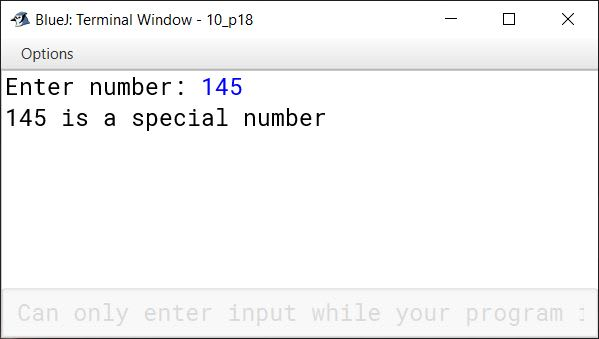#### Question 19

Write a menu driven program to accept a number and check and display whether it is a Prime number or not OR an Automorphic Number or not. (Use switch-case statement).

1. Prime number: A number is said to be a prime number if it is divisible only by 1 and itself and not by any other number.
Example: 3, 5, 7, 11, 13 etc.
2. Automorphic number: An automorphic number is the number which is contained in the last digit(s) of its square.
Example: 25 is an automorphic number as its square is 625 and 25 is present as the last two digits.

```import java.util.Scanner;

public class KboatPrimeAutomorphic
{
public static void main(String args[]) {
Scanner in = new Scanner(System.in);
System.out.println("1. Prime number");
System.out.println("2. Automorphic number");
int choice = in.nextInt();
System.out.print("Enter number: ");
int num = in.nextInt();

switch (choice) {
case 1:
int c = 0;
for (int i = 1; i <= num; i++) {
if (num % i == 0) {
c++;
}
}
if (c == 2)
System.out.println(num + " is Prime");
else
System.out.println(num + " is not Prime");
break;

case 2:
int numCopy = num;
int sq = num * num;
int d = 0;

/*
* Count the number of
* digits in num
*/
while(num > 0) {
d++;
num /= 10;
}

/*
* Extract the last d digits
* from square of num
*/
int ld = (int)(sq % Math.pow(10, d));

if (ld == numCopy)
System.out.println(numCopy + " is automorphic");
else
System.out.println(numCopy + " is not automorphic");
break;

default:
System.out.println("Incorrect Choice");
break;
}
}
}```
##### Output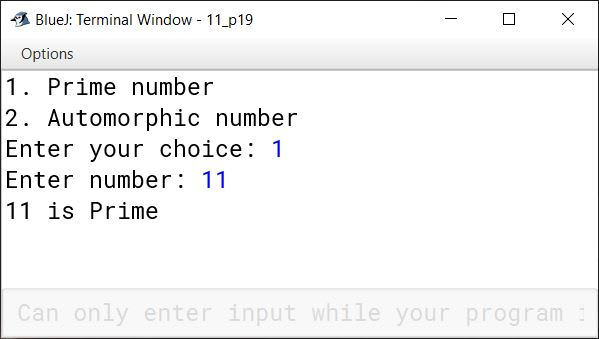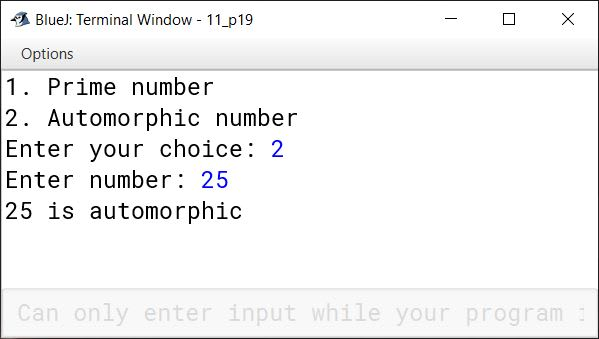#### Question 20

Write a menu driven program to access a number from the user and check whether it is a BUZZ number or to accept any two numbers and to print the GCD of them.

1. A BUZZ number is the number which either ends with 7 or is divisible by 7.
2. GCD (Greatest Common Divisor) of two integers is calculated by continued division method. Divide the larger number by the smaller; the remainder then divides the previous divisor. The process is repeated till the remainder is zero. The divisor then results the GCD.

```import java.util.Scanner;

public class KboatBuzzGCD
{
public static void main(String args[]) {
Scanner in = new Scanner(System.in);
System.out.println("1. Buzz number");
System.out.println("2. Calculate GCD");
int choice = in.nextInt();

switch (choice) {
case 1:
System.out.print("Enter a number: ");
int num = in.nextInt();
if (num % 10 == 7 || num % 7 == 0)
System.out.println(num + " is a Buzz Number");
else
System.out.println(num + " is not a Buzz Number");
break;

case 2:
System.out.print("Enter first number: ");
int a = in.nextInt();
System.out.print("Enter second number: ");
int b = in.nextInt();
while (b != 0) {
int t = b;
b = a % b;
a = t;
}
System.out.println("GCD = " + a);
break;

default:
System.out.println("Incorrect Choice");
break;
}
}
}```
##### Output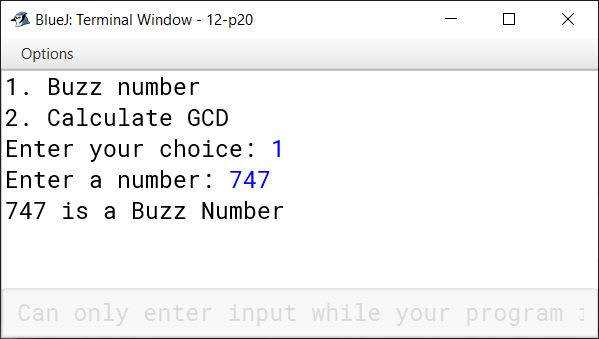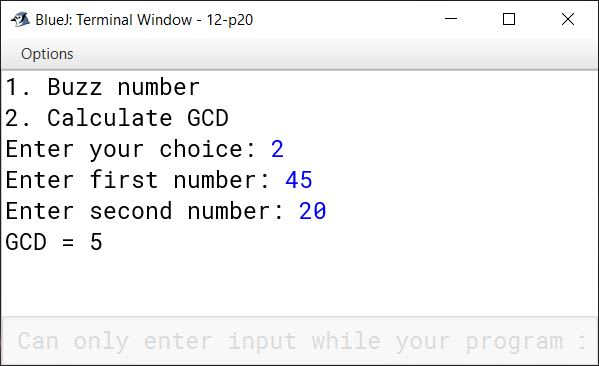#### Question 21(i)

Write a program in Java to find the sum of the given series:

x1 + x2 + x3 + x4 ... + xn

```import java.util.Scanner;

public class KboatSeries
{
public static void main(String args[]) {
Scanner in = new Scanner(System.in);
System.out.print("Enter x: ");
int x = in.nextInt();
System.out.print("Enter n: ");
int n = in.nextInt();
long sum = 0;
for (int i = 1; i <= n; i++)
sum += Math.pow(x, i);
System.out.println("Sum = " + sum);
}
}```
##### Output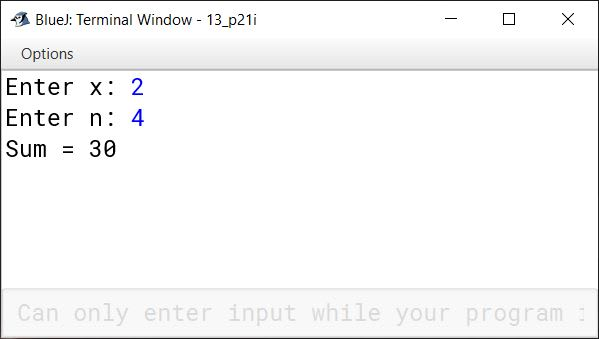#### Question 21(ii)

Write a program in Java to find the sum of the given series:

x1 - x2 + x3 - x4 ... - xn , where x = 3

```import java.util.Scanner;

public class KboatSeries
{
public static void main(String args[]) {
Scanner in = new Scanner(System.in);
System.out.print("Enter n: ");
int n = in.nextInt();
int x = 3;
double sum = 0;

for (int i = 1; i <= n; i++) {
double term = Math.pow(x, i);
if (i % 2 == 0)
sum -= term;
else
sum += term;
}
System.out.println("Sum = " + sum);
}
}```
##### Output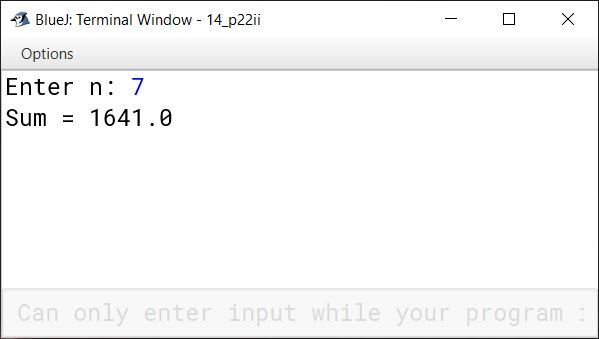#### Question 21(iii)

Write a program in Java to find the sum of the given series:

1/x1 + 2/x2 + 3/x3 + ... + n/xn

```import java.util.Scanner;

public class KboatSeries
{
public static void main(String args[]) {

Scanner in = new Scanner(System.in);
System.out.print("Enter x: ");
int x = in.nextInt();
System.out.print("Enter n: ");
int n = in.nextInt();
double sum = 0;

for (int i = 1; i <= n; i++) {
sum += (i / Math.pow(x, i));
}

System.out.println("Sum=" + sum);
}
}```
##### Output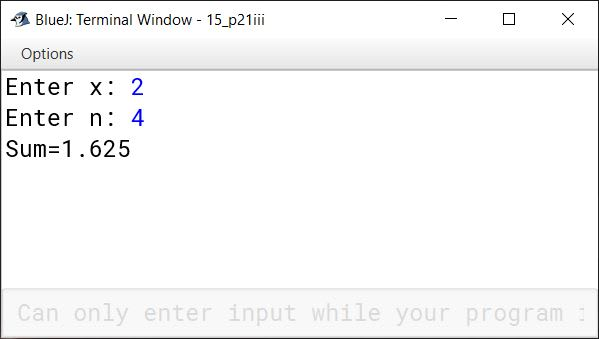#### Question 21(iv)

Write a program in Java to find the sum of the given series:

1/2 + 2/3 + 3/4 + ... + 49/50

```import java.util.Scanner;

public class KboatSeries
{
public static void main(String args[]) {
double sum = 0;
for (int i = 1; i <= 49; i++)
sum += i / (double)(i + 1);
System.out.println("Sum = " + sum);
}
}```
##### Output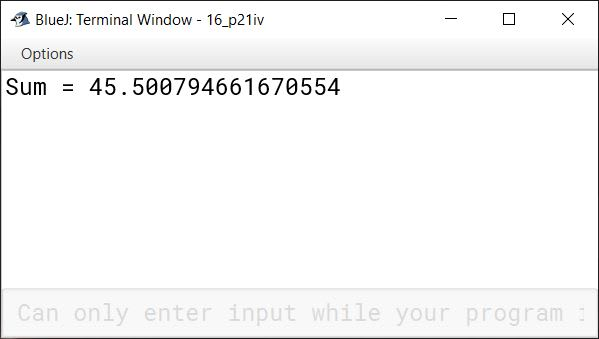#### Question 21(v)

Write a program in Java to find the sum of the given series:

1/√1 + 2/√2 + 3/√3 + ... + 10/√10

```public class KboatSeries
{
public static void main(String args[]) {
double sum = 0;
for (int i = 1; i <= 10; i++)
sum += i / Math.sqrt(i);
System.out.println("Sum = " + sum);
}
}```
##### Output